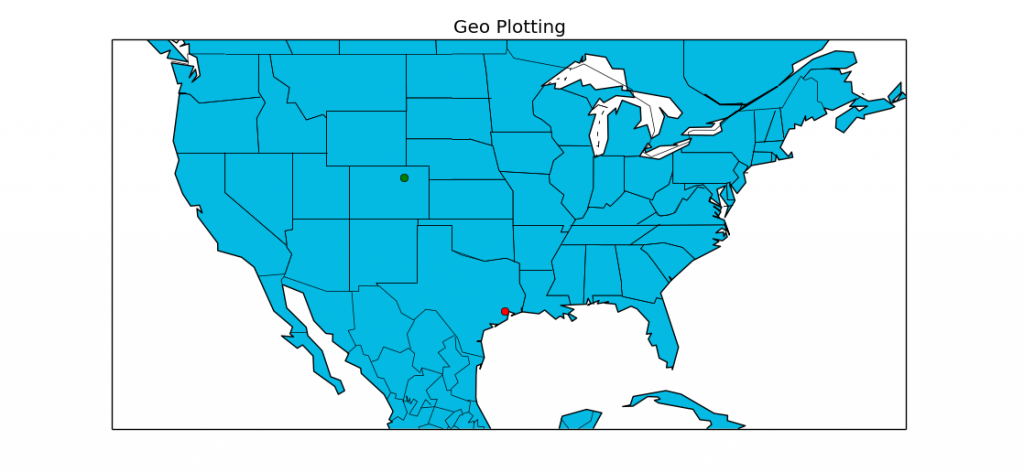## Geographical Plotting with Basemap and Python p. 4

In this tutorial with Basemap, you are shown how to actually plot a plot, as well as choose a zoom level on your projection. As you can see, plotting lat and long coordinates is fairly simple if you envision them as X and Y on a plane. The only confusing part is that X, Y translates to Lon, Lat... which is the reverse of how they are normally reported.

From the video, here is the sample code:

```from mpl_toolkits.basemap import Basemap
import matplotlib.pyplot as plt

def mapTut():

m = Basemap(projection='mill',llcrnrlat=20,urcrnrlat=50,\
llcrnrlon=-130,urcrnrlon=-60,resolution='c')
m.drawcoastlines()
m.drawcountries()
m.drawstates()
m.fillcontinents(color='#04BAE3',lake_color='#FFFFFF')
m.drawmapboundary(fill_color='#FFFFFF')

# Houston, Texas

lat,lon = 29.7630556,-95.3630556
x,y = m(lon,lat)
m.plot(x,y, 'ro')

lon, lat = -104.237, 40.125 # Location of Boulder

xpt,ypt = m(lon,lat)
m.plot(xpt,ypt, 'go')

plt.title("Geo Plotting")
plt.show()

mapTut()
```
The above code will generate a map in Matplotlib and Basemap with coordinates plotted for Houston TX and Boulder CO.

The next tutorial:• Matplotlib Crash Course

• 3D graphs in Matplotlib

• 3D Scatter Plot with Python and Matplotlib

• More 3D scatter-plotting with custom colors

• 3D Barcharts

• 3D Plane wireframe Graph

• Live Updating Graphs with Matplotlib Tutorial

• Modify Data Granularity for Graphing Data

• Geographical Plotting with Basemap and Python p. 1

• Geographical Plotting with Basemap and Python p. 2

• Geographical Plotting with Basemap and Python p. 3

• Geographical Plotting with Basemap and Python p. 4
• Geographical Plotting with Basemap and Python p. 5

• Advanced Matplotlib Series (videos and ending source only)